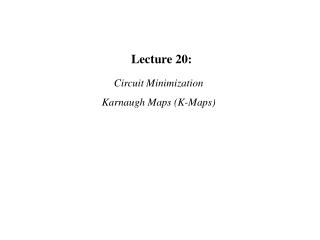DownloadDownload PresentationLecture 20:

# Lecture 20:

Télécharger la présentation## Lecture 20:

- - - - - - - - - - - - - - - - - - - - - - - - - - - E N D - - - - - - - - - - - - - - - - - - - - - - - - - - -
##### Presentation Transcript

1. Lecture 20: Circuit Minimization Karnaugh Maps (K-Maps)

2. The Relationship Between Venn Diagrams and Karnaugh Maps

3. Examples of Simplifying Groups of Terms in K-Maps

4. Karnaugh Maps (K-Maps) We will use a K-map to simplify the follwing expression. F(x,y,z) = xy’z’ + x’y’z + xz’ + y’z + x’ First we note that there are three variables in the expression F( ), therefore our K-map will have 23=8 cells. Next we decide where each variable will be represented. yz x

5. yz 00 01 11 10 x 0 1 yz 00 01 11 10 x 1 1 1 1 0 1 1 1 1 Now we arrange the literal values for x,y and z so that binary vectors that are next to each other differ by only one bit. In other words we make sure that logically adjacent vectors are also physically adjacent. Now we place ones (true values) into the K-map to show which binary vectors satisfiy the expression F( ). F(x,y,z) = xy’z’ + x’y’z + xz’ + y’z + x’ 100 001 1-0 100 110 -01 001 101 0-- 000 001 010 011

6. yz yz yz 00 01 11 10 00 01 11 10 00 01 11 10 x x x 1 1 1 1 1 1 1 1 1 1 1 1 0 1 0 1 0 1 1 1 1 1 1 1 1 1 1 Now we find the smallest number of the largest rectangular patterns of 1’s whose sizes are integer powers of two. (Weird) That is the length of a selected rectangle of ones must be of length 1,2,4,. . .,2n. Of course in this case 4 is the limit. We must choose a set of these rectangles such that every 1 is in at least one of the rectangles. Here are a few candidates. In each case we have contained all the 1’s in three rectangles of the required dimensions. The middle case is better because it uses larger patterns. Finally we convert these pattern back into terms in the simplified expression for F( ). F(x,y,z) = x’ + y’ + z’

7. Comparing K-Map and Algebraic Simplification

8. There are Many Equivalent K-Maps Configurations

9. Examples of 4-Variable K-Maps

10. s = a b cin. + + Simplifying the Full Adder Circuit Cout = ab + acin + bcin

11. A K-Map "Tiles the Plane" These terms are logically adjacent even though they appear on opposite corners of the K-Map

12. Sample Problems for Transforming Boolean Expressions to Other Equivalent Forms Note: For those of you who are unfamiliar with the conversions between SOP, POS, minterms and maxterms please work the following exercises as practice. If you have any questions please feel free to ask in class or contact me directly. Sum-of-Products Product-of-Sums minterms Maxterms ABC+AB’C+A’B’C’ (P+Q’+R)(P’+Q+R’) f(x,y,z)=m(0,1,3,4,6) g(a,b,c,d)=M(0,11,15) For each row, use the representation of the Boolean expression given to derive the other representations. Some representations will be too large to fit conveniently in the table.

13. Summary • The Relationship Between Venn Diagrams and Karnaugh Maps • Examples of Simplifying Groups of Terms in K-Maps • Karnaugh Maps (K-Maps) • Comparing K-Map and Algebraic Simplification • There are Many Equivalent K-Map Configurations • Examples of 4-Variable K-Maps • The K-Map"Tiles the Plane" • Max-Terms (Zeros) Can Be Used to Populate a K-Map • Sample Problems for Transforming Boolean Expressions • Sum-of-Products • Product-of-Sums • Minterms • Maxterms